Reach Us+447480022765
A Model of Light-Activated Changes in Reaction Centers of Purple Bacteria | OMICS International
Journal of Bioprocessing & Biotechniques

Like us on:

All submissions of the EM system will be redirected to Online Manuscript Submission System. Authors are requested to submit articles directly to Online Manuscript Submission System of respective journal.

A Model of Light-Activated Changes in Reaction Centers of Purple Bacteria

 MA Zabolotny1, YM Barabash2, Kulish MP1, Dmytrenko OP2 and Ramazanov DМ1* 1T Shevchenko Kyiv National University, 64, Volodymyrska Street, Kyiv 01033, Ukraine 2Institute of Physics of the NAS of Ukraine, 46, Prospect Nauky, Kyiv 03650, Ukraine Corresponding Author : Ramazanov DМ T Shevchenko Kyiv National University 64, Volodymyrska Street, Kyiv 01033, Ukraine Tel: 380-44-239-333 E-mail: [email protected] Received December 25, 2014; Accepted February 11, 2015; Published February 13, 2015 Citation: Zabolotny MA, Barabash YM, Kulish MP, Dmytrenko OP, Ramazanov DМ (2015) A Model of Light-Activated Changes in Reaction Centers of Purple Bacteria. J Bioprocess Biotech 5:208. doi: 10.4172/2155-9821.1000208 Copyright: © 2015 Zabolotny MA, et al. This is an open-access article distributed under the terms of the Creative Commons Attribution License, which permits unrestricted use, distribution, and reproduction in any medium, provided the original author and source are credited.

Visit for more related articles at Journal of Bioprocessing & Biotechniques

Abstract

We have studied the slow dynamics of isolated complexes of chlorophyll-containing membrane proteins of photosynthetic Reaction Centers (RCs) of Rb. sphaeroides R-26 induced by light fluxes. The analysis of a variation of the absorption of a solution of RCs in the frame of the three-level model is carried out. The equation defining the ratio of the populations of the electron levels of the primary and secondary quinones is deduced. The solution of this equation has non-Boltzmann character even in the stationary case and depends on the exciting light intensity. A model of the dynamics of levels of RC which accounts the presence of polarization processes in the vicinity of the secondary quinone acceptor (QB) is proposed. It is assumed that all RCs have the same structure but can be in different states, whose characteristics depend on both the time interval having passed after the absorption of a light quantum and the local viscosity and elasticity of the environment of QB. The presence of deformational properties of the environment of QB yields the possibility for a configuration of RC to be changed, which agrees with the experimental data.

 Keywords Biophysics; Bacterial reaction centers; Electron kinetics; Models Introduction It is known that the absorption of a quantum of light in the Reaction Centers (RCs) of purple bacteria Rb. sphaeroides R-26 causes the photo oxidation of the primary donor of an electron, a bacteriochlorophyll dimer (Ð ). Thereafter the excited electron appears at the primary (QÐ�) quinone acceptor, passes through a number of intermediate carriers, and then gets into the terminal quinone acceptor (QB) [1-3]. The process of localization of an electron at quinone acceptors is defined by the state of intraprotein hydrogen bonds (which are formed, in particular, with participation of water molecules of a solution) and the protonation of the nearest amino acid residues by ionogenic groups and is accompanied by a conformational rearrangement of the molecular complex of RC [2-5]. The information about regularities defining the character of light-activated structural transitions of biomolecules is of independent interest. The conformation determined the bioactivity of a molecule, and the control over structural transitions opens a possibility to use them in molecular electronics. Despite the great number of publications devoted to the study of photo induced processes running in RCs, there are the great numbers of obscurities in their theoretical comprehension. Far from being completed is the development of a model allowing the adequate description of the intramolecular dynamics of an electron under the action of exciting light. The complications arising in this case are caused by both the great number of stages of the process of electron transfer inside the complex of RC and the variety of the transition channels for an electron inside RC. In the simple case, it is considered that RC can be represented in the form of a protein matrix containing some builtin molecules (redox-cofactors) which manifest the donor-acceptor properties relative to the light-excited electron. The electron leaves the donor, a bacteriochlorophyll dimer, appears at the primary quinone QÐ�, passes a distance of 30-40 Å through a number of intermediate states, and is stabilized at the secondary quinone-acceptor QB, by generating, in this case, the difference of potentials on a photosynthetic membrane. The displacement of the electron induced by exciting light can be described  in the frame of the three-level model with the level of acceptor QB which slowly varies in the process of electron transfer. A change of the energy level of QB is defined by structural transitions in RC and polarization processes in its environment. A change of the energy characteristics of RC must lead to a change of the rate constants of photo induced electron transitions. At the present time, the kinetics of these most important characteristics of RC is studied slightly. The posed problem [2,5] concerning the electron transport in RC is complicated, and therefore the additional simplifying assumptions are used in its analysis [2,5]. Two of such assumptions are the quasiequilibrium behavior of the ratio of the electron populations on quinones QA and QB and the independence of this ratio on the intensity of exciting light. The conditions for these assumptions to be valid require the additional analysis. A goal of the present work is the development of a model of the processes defining structural photo induced changes in the molecular complex of RC. It is assumed that all molecules of RCs are the same and differ from one another only by the stages of light-stimulated changes. We took the influence of the intensity light on the ratio of the electron populations of Ð , QA, and QB into account. As the objects of investigations, we chose the transition rate constants in RC. The Three-Level Model of the Dynamics of an Electron in RC The structural transitions can be registered either directly (on the use of X-ray diffraction or photoluminescence analysis) or moderately with the help of interferometric, photoacoustic, or optical methods [2,5-7]. In what follows, we will analyze only the questions concerning the dynamics of electron transport in RC by studying the dynamics of the optical absorption spectrum at the wavelength λ0=865 nm [1- 7]. The possibility of such an approach is caused by the dependence of the interaction cross-section of photons with wavelength λ0 on the electronic state of the complex of RC which is changed under the action of the illumination of a specimen containing RCs by exciting light with intensity Ð� and wavelength λ0. When the electron leaves the bacteriochlorophyll dimer, the RC losses the ability to absorb the light with wavelength λ0. In this connection, a change of the absorption (ΔÐ�) of a specimen is proportional to a change of the probability p(t) of the presence of an electron on the bacteriochlorophyll dimer [2,5] at the time moment of the observation t: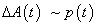(1) Relation (1) implies  that the rate constant (k21) of the return of electrons onto Ð  (donor) from acceptors (QA, QB) can be determined by the relation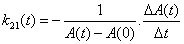(2) We now write the system of equations  describing the electron transfer in such a system. By QÐ�(t), we denote the probability of the presence of an electron on the primary quinone acceptor QA; and let QÐ�(t) be the probability of the presence of an electron on the terminal quinone acceptor QB. Then the following relations are valid: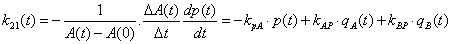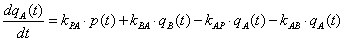(3)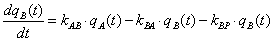Here, kij is the transition rate constant between the levels i→j. The processes running in the molecule of RC are initiated by exciting light, and therefore the parameter kÐ Ð� depends (is proportional to) on the intensity of exciting light I. The system of equations (3) should be supplemented by the relation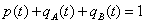(4) testifying to the closedness of the RC system. Values of the quantities kij (except for kÐ Ð�) for RC are given in the literature [2,5]. It is known that the transition rate constants have the following orders of magnitude: kÐ�Ð  ~ 10 s-1, kÐ�Ð  ~ (0.02 – 0.2) s-1, kAB ~ 104 s-1, kBA~ 103 s-1. The analysis of the system of equations (3) (without its solution) allows us to obtain the essential information significant for the comprehension of specific features of the functioning of RCs. We now determine the ratio of the populations of an electron on QÐ� and QB, namely,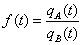(5) The use of this quantity allows us to write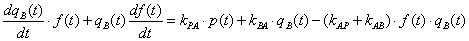(6) Substituting the last of Equations (3) in (6), we get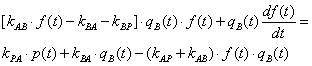(7) which yields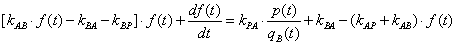(8) It follows from (8) that, even in the stationary case, the ratio of the electron populations of the levels of QB and QÐ� in the presence of the exciting illumination is not described by the Boltzmann relation but depends on the intensity Ð�. The ratio of the populations of an electron at QÐ� and QÐ� acquires an especially simple form in the absence of the exciting light (kPA = 0). Then we have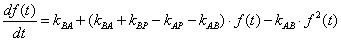(9) In order to solve Equation (8), it is necessary to set a value of f(t) at a certain (initial) time moment. It is natural to choose it as the time moment of the switching-out of light. Therefore, in the region of transient time moments, the function f(t) depends on the exciting light intensity even when the illumination is switched-out. Equation (9) is the exact consequence of the equations of balance (3) of the threelevel system and the condition of closedness of system (4). The analysis performed by us with the account of the above-presented transition rate constants showed that if we do not consider the time interval (0-10-3 ) s, then Equation (9) can be replaced by its stationary linear version,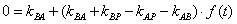(10) which yields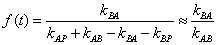(11) Relation (11) coincides with that in , but it can be used only in the study of the processes running in RC when the illumination is switched-out. While deducing the approximate relation (11), we used the above-given data on the transition rate constants for RC. The use of (11) allows us to write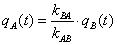(12) We now use (12) in the analysis of both Equation (4) and the first equation in (3) written in the case where the exciting light is absent (kÐ Ð� =0). Then we get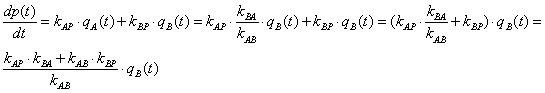(13)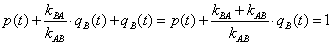(14) We now account that RCs are characterized by kAB ~ 104 s-1 >> kBA~ 103 s-1. Hence, relation (14) can be replaced by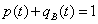(15) which allows us to write Equation (13) in the form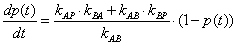(16) Equation (16) together with the initial condition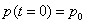(17) describes the kinetics of the RC system after the switching-out of exciting light. The parameter Ñ�0 in (17) is determined by the population of the donor of electrons at the time moment of the switching-out of light. A relation (16) and (17) gives a possibility to describe RC as a two-level system on the time intervals more than 0.001 s. In this case, the rate constant k21 of the transition of an electron from the acceptor (which is a dynamical combination of QA and QB-level 2) to donor Ð  (level 1) is determined by the relation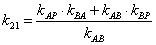(18) Thus, while studying the relaxation dynamics of the absorption of a solution of RCs to within the times of at least 0.001 s, we can use the equation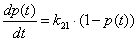(19) The rate constant of the return of an electron to the donor, k21, is given by relation (18). As the initial condition, we use representation (17). Thus, the quantities k21 and Ñ�0 are the unique characteristics which can be determined from the experiments [see formula (2)] on studying the relaxation of the absorption of a solution of RCs which arises after the termination of its exposure with exciting light. The repetition of the executed analysis in the case where Ð� ≠ 0 shows that the kinetics of the electron population of Ð  can be described by the equation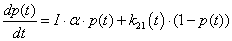(20) Where α is the coefficient of extinction which is practically independent of I in the experimentally studied interval of light intensities (at most 20 W/m2). In this case, the coefficient k21 should be determined with the help of Equation (8). We also additionally assumed [5,7] that the coefficient of extinction does not depend on time. Thus, specific features of the kinetics of RC are described in the model under consideration with the help of the modeling of the rate of inverse transitions. We assume that this quantity depends on time. Hence, we consider k21(t). The processes running in the RC complex on the switching-out of light are described by Equation (19), for which the initial condition takes the form(21) Where the parameter Ñ�0 describes the probability of the presence of electrons at the donor at the time moment of the switching-out of exciting light and depends on its intensity, the dependence of the exciting light intensity on time, and the temperature of a specimen. Specimens, Results of Measurements, and their Discussion In the present work, we used the isolated protein-pigment complexes (RCs) separated at the Chair of Biophysics of Lomonosov Moscow State University from the photosynthetic membranes of Rhodobacter sphaeroides cells by using a detergent lauryldimethylaminooxide (LDAO). To ensure the long-term stability of the parameters of RC, we used a water solution of 0.01 Ð� sodium-phosphate buffer at pH 7.2 with the addition of 0.05% detergent. The measuring cuvette was 3 × 5 × 2.5 cm in size with quartz walls of 1 mm in thickness. The solution of RCs with a concentration of ∼10-6 Ð� was held in dark at room temperature for at least 12 h (dark-adapted state). The light-adapted state was formed under illumination of the solution of RCs with a light pulse with varying duration and intensity [1-7]. The spectral range of an exciting light-emitting diode overlapped the most part of the absorption band of the RC «antenna». The relative number of centers and the transition rate from one state to another one were determined from the kinetics of the optical absorption spectrum at λ0= 865 nm with the use of Equation (19). The results of measurements are illustrated by Figures 1 and 2, where we show the recovery kinetics of the absorption of RCs after the switching-out (t=0) of exciting light (865 nm) with various intensities and durations. It is seen from the data that, after the switching-out of exciting light, the absorption of RCs approaches a stationary regime in 10 s. The jump of the values (Ð�(t = 0)÷Ð�(t > 10 s)) increases with the exposure of RCs. The results of processing of experimental data with the use of relation (18) are given in Figure 2, where the kinetics of the relaxation rate constant k21(t) of RCs to the dark-adapted state after the switchingout of exciting light with various intensities and durations is presented. This allows us to draw the following conclusions: 1) For the first 0.1 ÷ 2 s, the value of k21(t) is maximal and slightly varies in the course of time. After the termination of this time interval, the value of k21(t) decreases by 2 ÷ 3 orders for ~ 1 ÷ 3 s and then remains constant, k21. This fact testifies to the invariability of properties of RCs in this time interval; 2) An increase of the exposure duration of a specimen by exciting light or an increase of its intensity leads to a decrease of the parameter k21. Such a dependence of the relaxation rate constant k21(t) of RCs on time can be a consequence of the peculiarities of polarization effects in the vicinity of QÐ� due to viscoelastic properties of the environment. In order to obtain the information directly about the transition rate constants in RCs with the help of experimental data on k21(t), we use formula (18) and the assumption that the transition of an electron from QÐ� directly onto donor Ð  is strongly suppressed, and therefore we can set kÐ�Ð =0. In this case, the following relation is true: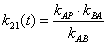According to the literature data [4-7], the rate constant kÐ�Ð  does not depend on time, and therefore the kinetics of k21(t) is completely determined by the rate constants kBA and kAB of the transitions of an electron between quinones QA and QB. Model for k21(t) in the Dark State To explain the indicated peculiarities of the behavior of k21(t), we propose a model which accounts the established relations between experimentally measured quantities and is schematically presented in Figure 3. In this figure, we also show the potential energies of the quinone molecules QA and QB, between which the electron transfer is realized. The abscissa axis is the generalized nuclear coordinate (Ñ�) describing the position of the nuclei of atoms belonging to the composition of the corresponding quinone. During the presence of an electron at a quinone, the local electronic and orientational components of the polarization of the environment are created under the action of the electric field. These components determine the potential energy of the corresponding quinone . The high-frequency component of the electronic polarization of the environment with frequency greater than the characteristic one of the electron transfer goes automatically after the electron under its transfer. Therefore, on the electron transfer, the orientational polarization of the environment different from the previous one must appear in the vicinities of QA and QB. In accordance with the Marcus model [2,8], we assume that the polarization of the environment in the initial state can be randomly varied and shifted in the course of the fluctuation motion of the nuclei of quinone molecules to the direction of the polarization corresponding to the reaction products. This changes the potential energy of the corresponding quinones and allows the electron transfer to occur. The energy profile in Figure 3 arises due to the quasi intersection of two potential curves corresponding to the orientational ordering of molecules of the environment around the initial complex and the reaction products. If the system attains the point where the potential curves intersect, there occurs the instantaneous electron transfer. Let the potential energy UÐ�(y) of quinone QA attain the minimum at the point Ñ�=0. For simplicity, we assume that the function UÐ�(y) has [2,5,9] parabolic character: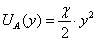(22) Here, χ is the parameter which defines the steepness of branches of the potential UÐ�(y) and depends on the parameters defining the dynamics of polarization processes (e.g., on local rheological characteristics of the environment). We assume that the potential energy UÐ�(y) of quinone QB is described by a formula analogous to (22) with regard for the fact that the nuclear coordinate of the secondary quinone is shifted and is located at the point we denote as yÐ�: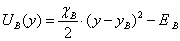(23) The sense of the parameter χÐ� is analogous to that of χ. The quantity Ð�Ð� sets the minimum value of the potential energy of the QB molecule. We also assume that the nearest environment of QB is not toughly fixed , and, on the appearance of an electron on the secondary quinone, its polarization varies in time due to the Coulomb forces (Figure 4). This must imply that the quantities χÐ�, yÐ�, and Ð�Ð� turn out to be dependent on time. The assumption made is partially supported by experiments on the measuring of the volume of RCs present in the solution . Indeed, the volume of RCs decreases by 2 ÷ 4% under the action of light. For simplicity, we suppose that χ=χÐ� and only yÐ�(t) and Ð�Ð�(t) depend on time. As the important parameters of the model, we indicate the characteristics (yÐ¡(t), Ð�Ð¡(t)) of the point of quasi intersection of the curves UÐ�(y) and UÐ�(y).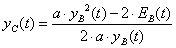(24)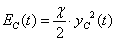(25) In the frame of the proposed model, the quantities yÐ¡(t) and Ð�Ð¡(t) reveal the nontrivial dependence on time caused by the polarization processes leading also to the dependences Ñ�Ð�(t) and Ð�Ð�(t). Let us assume  that the environment of QB is characterized by viscosity and elasticity. In this case, if an electron gets into QB (the probability of the presence of an electron is QB(t)), then there occurs a polarization of the environment under the action of the electric field of the electron, which changes its potential energy. We assume that the rate of variation of the potential energy of the electron is proportional to the probability of its presence at QB (by μ, we denote the corresponding coefficient of proportionality). Once the electron leaves the level of QB, it begins to relax to the initial state. We assume, first, that the rate of this process increases with a deviation of the level from its value Ð�Ð� 0 at the time moment of the switching-on of exciting light and, second, the characteristic duration of relaxation (τ) is independent of this quantity. In such a case, the equation describing the dynamics of level 2 takes the form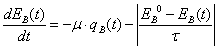(26) Equation (26) should be supplemented by the initial condition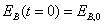(27) where Ð�B,0 is the value of the energy of QB at the time moment of the switching-out of exciting light (t=0). As for the possible dependence of yÐ�(t), we assume, following work and taking results in  into account, that the difference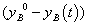is proportional to the quantity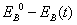. Here, yÐ� 0 is the coordinate of QB in the equilibrium dark-adapted state. In this case, the following relation is valid:(28) where α is the dimensional coefficient of proportionality. Thus, relations (22)-(28) define the model of the temporal evolution of the potential energy of quinone QÐ�. With the purpose to explain specific features of the kinetics of k21(t), we consider the peculiarities of the electron transitions QÐ�↔QÐ�. In Figure 3, we present the scheme of the potential energies of quinones QÐ� and QÐ�, by setting the characteristics of the electron transitions between them. Light is switched-out at the time moment t0=0, and the time t>t0corresponds to the case where RC is in dark. By assuming the activation mechanism of overcoming the barrier separating the energy levels of electrons at quinones QÐ� and QÐ� , we note that the value of k21 is determined by the height of this barrier Δ(t) according to the relation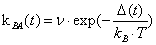(29) Here, ν is the frequency factor including the matrix element of the transition QÐ�→QÐ�, kB is the Boltzmann constant, and Ð¢ is the temperature of a specimen. If the electron overcomes the barrier by the tunneling mechanism, relation (29) should be replaced by the relevant one from work . The value of kÐ�Ð� is determined by the relation analogous to (29):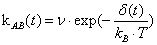(30) Here, the quantity δ (Figure 3) is the height of the energy barrier which is overcome by the electron on the transition from quinone QÐ� onto QÐ�. While deducing formulas (29) and (30), it was assumed that the electron transitions QÐ�↔QÐ� occur by the activation mechanism; and we also take, to simplify the consideration, that the hopping frequencies of the electron for the transitions QÐ�→QÐ� and QÐ�→QÐ� are the same. Relations (22), (29), and (30) yield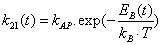(31) We recall that Ð�Ð�(t) is the depth of the potential well, in which the electron is located during its presence at QÐ�, relative to the minimally possible value of the energy of the electron positioned at quinone QÐ�. From relation (31), the experimental data given in figures 2-4, and the approximate relation kÐ�Ð  ~ 10 s-1, we can get the information about the kinetics of the electron level of QÐ� relative to the electron level of QÐ�: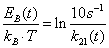(32) The temporal behavior of Ð�Ð�(t) following from the data given in Figure 2 is shown in Figure 4. Conclusion 1. We have established the essential dependence of the rate constant for the transition of the electron from the acceptor to the donor on time after the switching-out of exciting light. This dependence has the S-like behavior. Such a dependence of k21(t) can be explained by a distortion of the electron level of the terminal acceptor QÐ� as a result of polarization effects under multiple photo induced transitions of the electron in the RC macromolecule. 2. During the first 0.1 ÷ 2 s, the value of the transition rate constant of electrons is maximal and depends slightly on time. After this time interval, the constant decreases by 2 ÷ 3 orders for approximately 3 s. Then the value of the transition rate constant of electrons remains invariable, which testifies to the stability of properties of RCs in this time interval. 3. We have developed a theoretical model which describes the transition of electrons 2→1 with regard for the possibility for polarization processes to occur in the vicinity of level 2. These processes are running under the action of ponderomotive forces of the electric origin due to the field of the electron located on level 2. It is assumed that the peculiarities of the formation of a polarized environment of level 2 can be described with regard for its local viscosity and elasticity. The model consider the possibility for the distance between the levels to be changed on a variation of the polarization of level 2, which can be an analog of a variation of the conformational variable. The model explains qualitatively the experimental regularities of the relevant kinetics and describes specific features of the temporal variation of the rate constant for the transition of electrons from the acceptor onto the donor when the exciting light is switched-out. 4. We have found the unique combination of the transition rate constants for RC with the help of the analysis of the experimental data on the behavior of RCs after the switching-out of light and have determined the kinetics of the electron level of quinone QB relative to the level of quinone QÐ�. References Capaldi RA (1979)Membrane proteins in energy transduction. Marcel Dekker, New York, USA, 279-340. Rubin AB (2000) Biophysics. Moscow State University, Moscow, Russia 2. Amin M, Vogt L, Vassiliev S, Rivalta I, Sultan MM, et al. (2013)Electrostatic effects on proton coupled electron transfer in oxomanganese complexes inspired by the oxygen-evolving complex of photosystem II.J PhysChem B 117: 6217-6226. Kharkyanen VN, Barabash Yu M, Berezetskaya NM, Lukashev EP, Knox PP, et al. (2011) Peculiarities of light-induced slow protein dynamics in the photosynthetic reaction center. ChemPhysLett 512: 113-117. BarabashYu M, Berezetskaya NM, Christophorov LN, Goushcha AO, Kharkyanen VN(2002) Effects of structural memory in protein reactions. J ChemPhys116: 4339-4352. Kukushkin AK, Tikhonov AN (1988) Lecture on Biophysics of Photosynthesis. Moscow State University, Moscow BarabashIuM, ZabolotnyÄ­ MA, Sokolov NI, Kharkianen VN (2002) Slowphotoinduced changes in reaction centers of Rhodobactersphaeroides R-26 by holographic interferometry. Biofizika 47: 970-976. Gol’danskii VI, Trakhtenberg LI, Fleurov VN (1986) Tunneling Phenomena in Chemical Physics. Nauka, Russia. Goushcha AO, Kapoustina MT, Kharkyanen VN, Holzwarth AR (1997)Nonlinear Dynamic Processes in an Ensemble of Photosynthetic Reaction Centers. Theory and Experiment. JPhysChem B101: 7612-7619.

Figures at a glance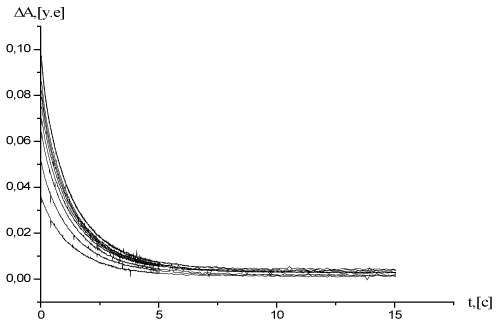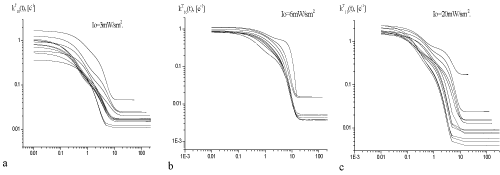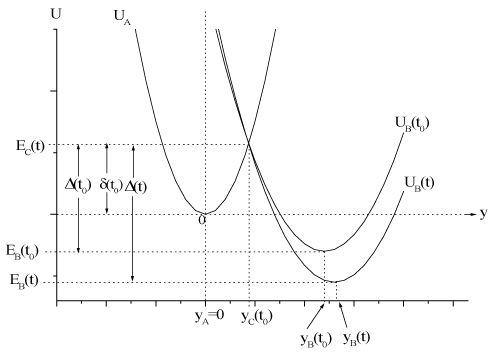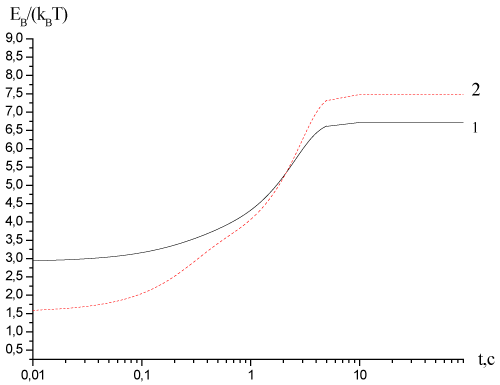Figure 1 Figure 2 Figure 3 Figure 4
Select your language of interest to view the total content in your interested language

Article Usage

• Total views: 11871
• [From(publication date):
February-2015 - Oct 23, 2019]
• Breakdown by view type
• HTML page views : 8103Can't read the image? click here to refresh